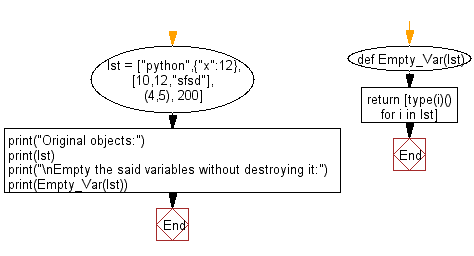﻿ Python: Empty a variable without destroying it - w3resource# Python: Empty a variable without destroying it

## Python Basic: Exercise-122 with Solution

Write a Python program to empty a variable without destroying it.

type() returns the type of an object, which when called produces an 'empty' new value.

Sample data: n=20
d = {"x":200}
Expected Output: 0
{}

Sample Solution-1:

Python Code:

``````n = 20
d = {"x":200}
l = [1,3,5]
t= (5,7,8)
print(type(n)())
print(type(d)())
print(type(l)())
print(type(t)())
```
```

Sample Output:

```0
{}
[]
()
```

## Visualize Python code execution:

The following tool visualize what the computer is doing step-by-step as it executes the said program:

Sample Solution-2:

Python Code:

``````def Empty_Var(lst):
return [type(i)() for i in lst]
lst = ["python",{"x":12},[10,12,"sfsd"], (4,5), 200]
print("Original objects:")
print(lst)
print("\nEmpty the said variables without destroying it:")
print(Empty_Var(lst))
```
```

Sample Output:

```Original objects:
['python', {'x': 12}, [10, 12, 'sfsd'], (4, 5), 200]

Empty the said variables without destroying it:
['', {}, [], (), 0]
```

Flowchart:## Visualize Python code execution:

The following tool visualize what the computer is doing step-by-step as it executes the said program:

Python Code Editor:

Have another way to solve this solution? Contribute your code (and comments) through Disqus.

What is the difficulty level of this exercise?

Test your Python skills with w3resource's quiz

﻿

## Python: Tips of the Day

Unpack variables from iterable:

```# One can unpack all iterables (tuples, list etc)
>>> a, b, c = 1, 2, 3
>>> a, b, c
(1, 2, 3)

>>> a, b, c = [1, 2, 3]
>>> a, b, c
(1, 2, 3)
```